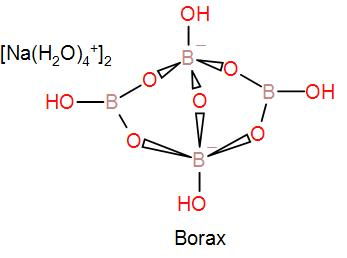QuestionAnswers

# The formula of mineral borax is:[A]$N{{a}_{2}}{{B}_{4}}{{O}_{7}}$[B]$N{{a}_{2}}{{B}_{4}}{{O}_{7}}\cdot 4{{H}_{2}}O$[C]$N{{a}_{2}}{{B}_{4}}{{O}_{7}}\cdot 5{{H}_{2}}O$[D]$N{{a}_{2}}{{B}_{4}}{{O}_{7}}\cdot 10{{H}_{2}}O$Verified
129.9k+ views
Hint:
Borax is a naturally occurring mineral. It contains sodium, boron, oxygen and hydrogen atoms. It is generally written in its octa-hydrate form but it is also written in its deca-hydrate form.

Borax is a naturally occurring mineral which is used for the preparation of boric acid. We also know borax as sodium borate. Borax is a white crystalline solid.
We use borax in many different ways. It is the main component of detergents and cosmetics too.
We also use borax in the detection of basic radicals, $C{{u}^{2+}},N{{i}^{2+}},M{{g}^{2+}}$.
Borax is naturally found as deposits by repeated evaporation of lakes in crystalline form.
The formula of mineral borax is $N{{a}_{2}}\left[ {{B}_{4}}{{O}_{5}}{{\left( OH \right)}_{4}} \right]\cdot 8{{H}_{2}}O$.
We can draw its structure as-In the mineral borax, two $B{{O}_{3}}$ and two $B{{O}_{4}}$ units form the close anionic structure found in borax. As we can see in the diagram of its structure, the two boron atoms are tetrahedrally coordinated and the other two boron atoms are in a trigonal planar environment.
Borax is a crystalline solid and it is soluble in water and it is easily converted to boric acid by addition of HCl.
The formula of borax is written as $N{{a}_{2}}\left[ {{B}_{4}}{{O}_{5}}{{\left( OH \right)}_{4}} \right]\cdot 8{{H}_{2}}O$ showing its water of crystallization content but we can also write it as $N{{a}_{2}}{{B}_{4}}{{O}_{7}}\cdot 10{{H}_{2}}O$.

Therefore, the correct answer is the option [D] $N{{a}_{2}}{{B}_{4}}{{O}_{7}}\cdot 10{{H}_{2}}O$.

Note:
As we have read above that borax is soluble in water due to this, it is found as an evaporite deposit. The term evaporite is used for water soluble mineral which is formed due to concentration and crystallisation by evaporation from an aqueous solution. Due to this, it is found as deposits of seasonal lakes.